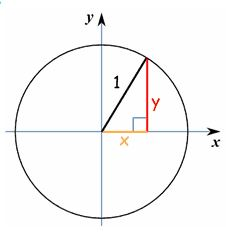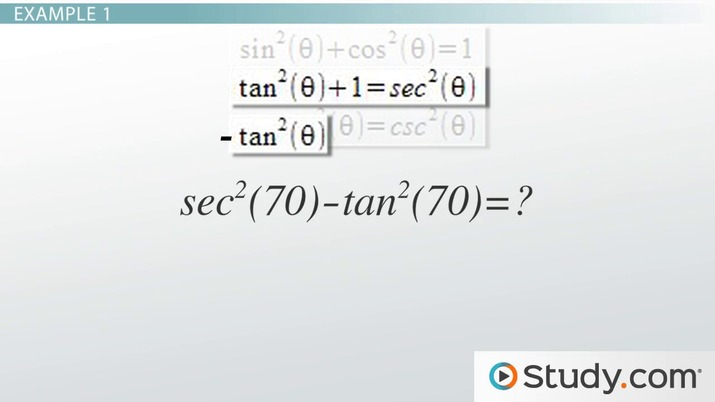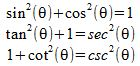Pythagorean Identities: Uses & Applications

Lesson Transcript
Instructor: Yuanxin (Amy) Yang Alcocer

Amy has a master's degree in secondary education and has been teaching math for over 9 years. Amy has worked with students at all levels from those with special needs to those that are gifted.

Pythagorean identities are trigonometric functions derived from the Pythagorean theorem. Learn about the uses and applications of Pythagorean identities, and solve trigonometric problems using Pythagorean identities. Updated: 10/19/2021

Pythagorean Identities

Today, we will look at our Pythagorean identities, which are a part of our trig identities. These Pythagorean identities are true statements about trig functions based on the Pythagorean theorem. You will see how they are based on the Pythagorean theorem (a2 + b2 = c2). If you look at the unit circle in trig and then look at the right triangle that is part of it, the two sides, a2 and b2, are our trig functions, while the hypotenuse, c, is the number 1.We have a total of three identities that are part of the Pythagorean identities. We have one that involves cosine and sine, another one that involves tangent and secant, and a third that involves cotangent and cosecant.An error occurred trying to load this video.

Try refreshing the page, or contact customer support.

Coming up next: Reciprocal Identities: Uses & Applications

You're on a roll. Keep up the good work!

Replay
Your next lesson will play in 10 seconds
• 0:02 Pythagorean Identities
• 1:34 Example 1
• 2:33 Example 2
• 3:55 Lesson Summary
Save Save

Want to watch this again later?

Timeline
Autoplay
Autoplay
Speed SpeedUses & Applications

Think of these identities as formulas that help you connect our trig functions. You can see that our trig functions are related by the Pythagorean theorem. These identities are also formulas that help us simplify our problems. You can see that if we have a function that is the sum of the square of a sine and the square of a cosine of the same angle, then we can simplify that expression as simply 1.

You will see these Pythagorean identities in use in problems that ask you to prove other trig statements as well as in simplifying more complex problems. Mathematicians also use the identities to help them figure out more complex problems using higher math, such as calculus.

Do you want to know what kinds of problems you can expect to see? Okay, let's look at a couple.

Example 1

Our first problem is asking us, 'What does sec2 (70) - tan2 (70) equal?'

At first glance, we might think that we need to calculate each function, square them, and then subtract them. But if we take a moment and think about our trig identities, in particular our Pythagorean identities, we will see that there is a much simpler, quicker, and more accurate way to solve our problem. We look through our identities and we see that our second one has both the tangent and secant functions in it.

It isn't written exactly like ours, but we do see that if we use our algebra skills and rearrange the identity a bit we will see that we can get our problem function. If we subtract the tangent from both sides, our identity becomes sec2 (theta) - tan2 (theta) = 1. This identity also gives us the answer to our problem. Do you see it? It's a nice, simple 1. Wasn't that easy?

Example 2

Let's look at one more problem.

To unlock this lesson you must be a Study.com Member.

Register to view this lesson

Are you a student or a teacher?